1. /
2. CBSE
3. /
4. Class 08
5. /
6. Mathematics
7. /
8. NCERT Solutions for Class...

# NCERT Solutions for Class 8 Maths Exercise 8.1### myCBSEguide App

Download the app to get CBSE Sample Papers 2023-24, NCERT Solutions (Revised), Most Important Questions, Previous Year Question Bank, Mock Tests, and Detailed Notes.

NCERT solutions for Class 8 Maths Comparing Quantities

## NCERT Solutions for Class 8 Maths Comparing Quantities

###### 1. Find the ratio of the following:

(a) Speed of a cycle 15 km per hour to the speed of scooter 30 km per hour.

(b) 50 m to 10 km

(c) 50 paise to Rs.5

Ans. (a) Speed of cycle = 15 km/hr

Speed of scooter = 30 km/hr

Hence ratio of speed of cycle to that of scooter = 15 : 30 =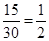= 1 : 2

(b)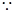1 km = 1000 m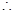10 km =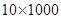= 10000 mRatio =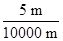=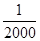= 1 : 2000

(c)1 = 100 paise5 =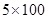= 500 paise

Hence Ratio =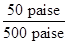=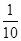= 1 : 10

###### 2. Convert the following ratios to percentages:

(a) 3 : 4

(b) 2 : 3

Ans. (a) Percentage of 3 : 4 =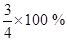= 75%

(b) Percentage of 2 : 3 =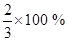=NCERT Solutions for Class 8 Maths Exercise 8.1

###### 3. 72% of 25 students are good in mathematics. How many are not good in mathematics?

Ans. Total number of students = 25

Number of good students in mathematics  = 72% of 25 =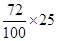= 18

Number of students not good in mathematics  = 25 – 18 = 7

Hence percentage of students not good in mathematics =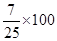= 28%

###### 4. A football team won 10 matches out of the total number of matches they played. If their win percentage was 40, then how many matches did they play in all?

Ans. Let total number of matches be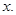According to question,

40% of total matches = 10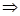40% of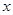= 10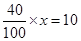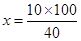= 25

Hence total number of matches are 25.

NCERT Solutions for Class 8 Maths Exercise 8.1

###### 5. If Chameli had Rs. 600 left after spending 75% of her money, how much did she have in the beginning?

Ans. Let her money in the beginning beAccording to question,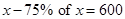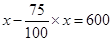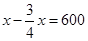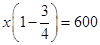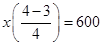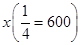=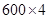= 2400

Hence the money in the beginning was `2,400.

NCERT Solutions for Class 8 Maths Exercise 8.1

###### 6. If 60% people in a city like cricket, 30% like football and the remaining like other games, then what percent of the people like other games? If the total number of people are 50 lakh, find the exact number who like each type of game.

Ans. Number of people who like cricket

= 60%

Number of people who like football

= 30%

Number of people who like other games

= 100% – (60% + 30%) = 10%

Now Number of people who like cricket

= 60% of 50,00,000

=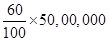= 30,00,000

And Number of people who like football

= 30% of 50,00,000

=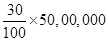= 15,00,000Number of people who like other games = 10% of 50,00,000

=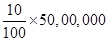= 5,00,000

Hence, number of people who like other games are 5 lakh.

## NCERT Solutions for Class 8 Maths Exercise 8.1

NCERT Solutions Class 8 Mathematics PDF (Download) Free from myCBSEguide app and myCBSEguide website. Ncert solution class 8 Mathematics includes text book solutions from Class 8 Maths Book . NCERT Solutions for CBSE Class 8 Maths have total 16 chapters. 8 Maths NCERT Solutions in PDF for free Download on our website. Ncert class 8 solutions PDF and Maths ncert class 8 PDF solutions with latest modifications and as per the latest CBSE syllabus are only available in myCBSEguide.

## CBSE app for Class 8

To download NCERT Solutions for class 8 Social Science, Computer Science, Home Science,Hindi ,English, Maths Science do check myCBSEguide app or website. myCBSEguide provides sample papers with solution, test papers for chapter-wise practice, NCERT solutions, NCERT Exemplar solutions, quick revision notes for ready reference, CBSE guess papers and CBSE important question papers. Sample Paper all are made available through the best app for CBSE students and myCBSEguide website.Test Generator

Create question paper PDF and online tests with your own name & logo in minutes.myCBSEguide

Question Bank, Mock Tests, Exam Papers, NCERT Solutions, Sample Papers, Notes

### 14 thoughts on “NCERT Solutions for Class 8 Maths Exercise 8.1”

1. Wow

2. its verry useful thank you

4. This is best
Shohib Aziz

5. good

6. Good guru g

7. Best teacher